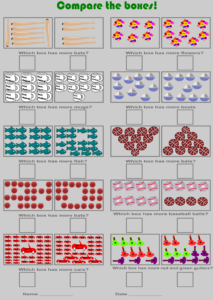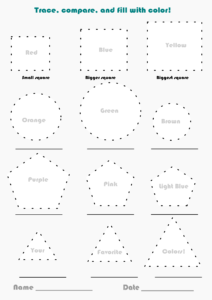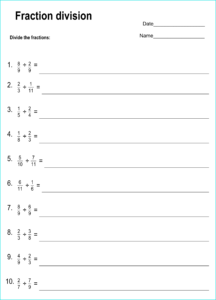## Printable dividing fractions worksheets with answers (PDF)

If you already checked out the worksheets we created with problems of adding and subtracting fractions, and multiplying fractions, then dividing fractions is the next step. They will have...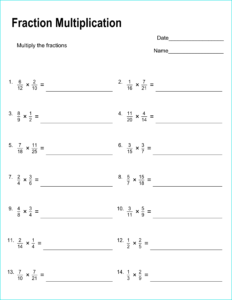## Free printable multiplying fractions worksheets with answers

Multiplying fractions can be a really hard concept for kids to grasp, which is why you have to offer them the proper tools and a lot of motivation by...## Free, printable decimal-to-fraction conversion chart

When kids reach 4th grade, they’re introduced to fractions and decimals in math, which means this printable decimal to fraction conversion chart can be very useful for them to...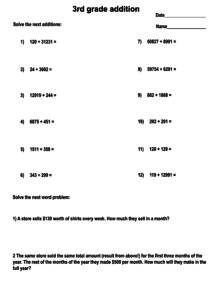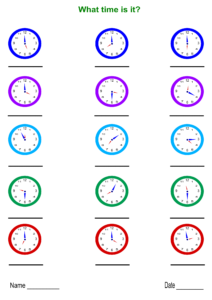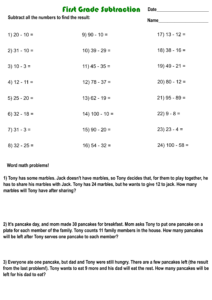Prepare your kid to face new math challenges in the first year of school with these 1st grade math worksheets. Help them ace through their new tests with the...